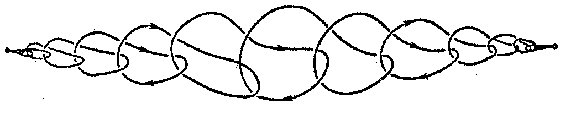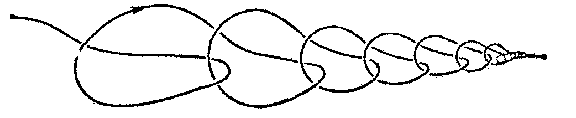# Wild sphere

A closed manifold in Euclidean three-space $E^3$ obtained by a wild imbedding of the sphere $S^2$ in $E^3$. Thus, a wild sphere is the sum of two discs with a common boundary, which is a wild knot. The first example of a wild sphere is the so-called "horned sphere of Alexanderhorned sphere" or Alexander sphere (Fig. a); it bounds a domain which is not homeomorphic to $E^3$ (in the figure this is the interior of the cylinder without any interlinking handles and points forming their boundary). Fig. b shows a wild sphere in which the exterior domain alone is not homeomorphic to $E^3$.